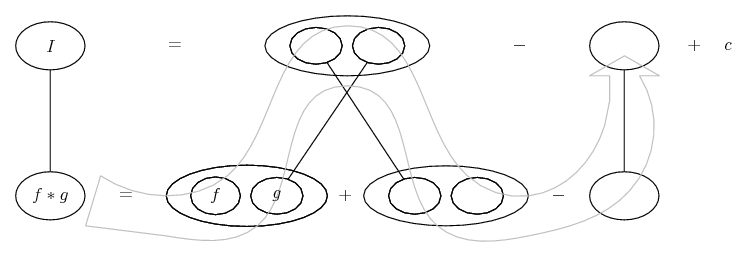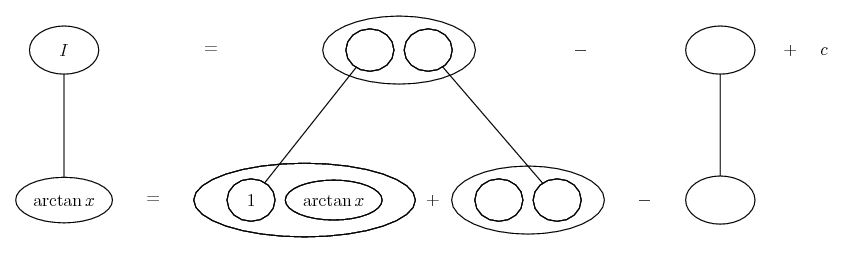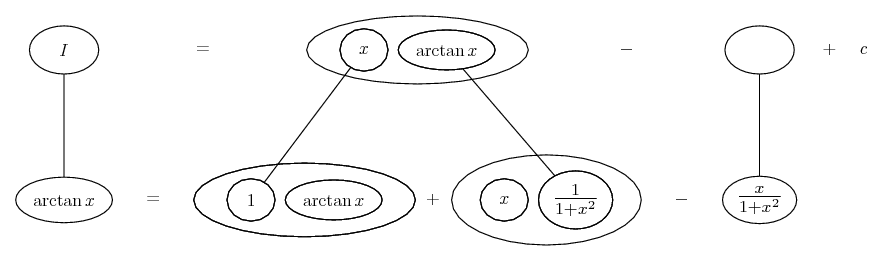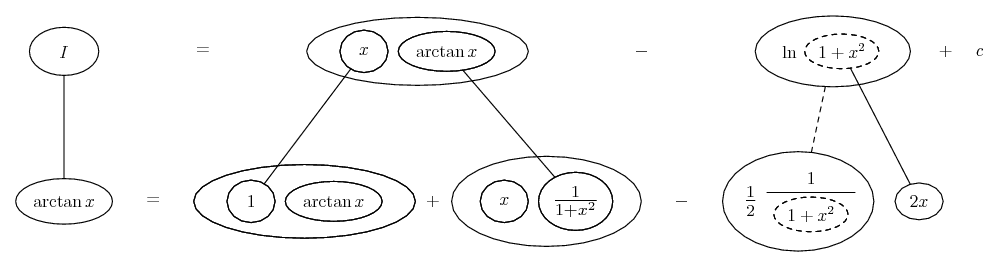1. ## integration

integral(tan(inverse) (2*x-1)/(1+x-x^2)) is

what should i do of tan(inverse) x is there any integration of it

2. ## Re: integration

With the substitution $\displaystyle 1+x-x^{2}= u$ the integral becomes...

$\displaystyle -\int \tan^{-1} u\ du$ (1)

Kind regards

$\displaystyle \chi$ $\displaystyle \sigma$

3. ## Re: integrationOriginally Posted by prasumwhat should i do of tan(inverse) x is there any integration of it
Yes - parts (after the sub given by chisigma).

Just in case a picture helps...... is lazy integration by parts, doing without u and v.

... where (key in spoiler) ...

Spoiler:... is the product rule, where straight continuous lines differentiating downwards with respect to x (or u, or whatever you've substituted for).

So you would have...(but with u, or whatever; these I made earlier.)

Spoiler:Spoiler:... where...
Spoiler:... is the chain rule. Straight continuous lines differentiate downwards (integrate up) with respect to the main variable (x, or whatever), and the straight dashed line similarly but with respect to the dashed balloon expression (the inner function of the composite which is subject to the chain rule).

__________________________________________________ __________

Don't integrate - balloontegrate!

Balloon Calculus; standard integrals, derivatives and methods

Balloon Calculus Drawing with LaTeX and Asymptote!

#### Search Tags

integration